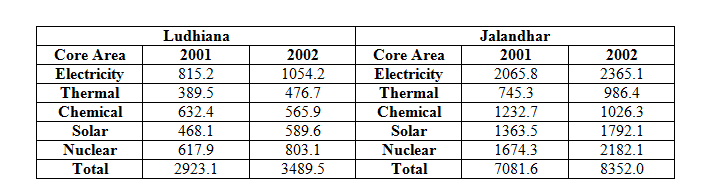Direction for questions 1 to 4:  Answer the questions based on the following information.
The amount of money invested (rupees in crores) in the core infrastructure areas of two districts, Ludhiana and Jalandhar in Punjab, is as follows.Question 1. By what per cent was the total investment in the two districts more in 2002 as compared to 2001?
a. 14%
b. 21%
c. 24%
d. 18%

Question 2. The investment in electricity and chemical energy in 2001 in these two districts formed what per cent of the total investment made in that year?
a. 41%
b. 47%
c. 52%
d. 55%

Question 3. In Jalandhar district, the investment in which area in 2002 showed the highest percentage increase over the investment in that area in 2001?
a. Electricity
b. Thermal
c. Solar
d. Nuclear

Question 4. Approximately how many times was the total investment in Ludhiana was to the total investment in Jalandhar?
a. 2.8
b. 2
c. 2.4
d. 1.7

Total investment in the two districts in year 2001 = 2932.1+7081.6 ≈ 10,000

Total investment in the two districts in year 2002 = 3489.5+ 8352 ≈ 11840

Required percentage = (11840 – 10000)/10000 = 18%(approx.)

From the given data we know that,

Total investment in electricity and chemical energy in both the districts in 2001 = (815.2 + 632.4 + 2065.8 + 1232.7) = 4746.1

Total investment made in that year = 2923.1 + 7081.6 = 10004.7 ≈ 10000

∴required percentage = (4746.1/10000) = 47% (approx.)

Percentage increase in investment in electricity = 300×100/2070 = 14%

Percentage increase in investment in thermal= {(986.4 – 745.3)/745.31} x 100 = 240/745 = 32%

Percentage increase in investment in solar

= (428.6×100/1363.5) let’s take the approximate values = 430/1360 = 31.5%

And the Percentage increase in investment in nuclear

= 507/1674 approximately = (500/1670)x 100 = 30%

So, clearly percentage increase in investment in thermal is the highest